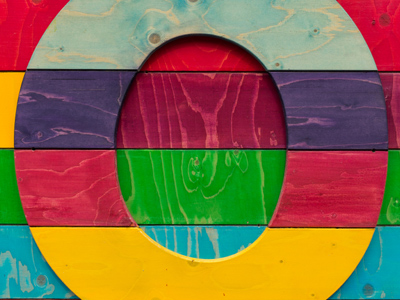All the numbers in the 10 times table end with a 0.

# Ten Times Table

In KS1 Maths and Numeracy children will be exposed to the times tables as early as possible in Year 1. The 1, 2, 5 and 10 times tables are taught first before the 3, 4, 6, 7, 8 and 9 times tables follow. By the end of Year 2 children are often fluent in their times tables up to 10.

This quiz will test children's familiarity with their ten times table, which will help them in studying maths in Years 1 and 2.

Do you know your ten times table? Let's find out!

1.
7 x 10 =
60
70
80
75
2.
2 x 10 =
12
15
20
5
3.
10 x 1 =
11
1
0
10
If a number is multiplied by 1 it will stay the same - even the number 10!
4.
10 x 0 =
100
1
10
0
Even 10 becomes 0 when it is multiplied by 0
5.
10 x 5 =
60
55
45
50
To times a number by 10 just add a 0 to the end of it
6.
4 x 10 =
40
30
50
35
7.
6 x 10 =
60
70
72
50
8.
3 x 10 =
35
40
30
32
9.
10 x 9 =
99
95
90
93
All numbers in the ten times table end with a 0
10.
10 x 10 =
90
100
95
99
100 is 10 lots of 10
Author:  Graeme Haw